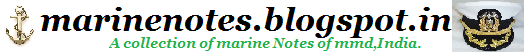Home » » Centrifugal Pump Fundamentals

# Centrifugal Pump Fundamentals

 Centrifugal Pump Fundamentals A-1 Head The pressure at any point in a liquid can be thought of as being caused by a vertical column of the liquid which, due to its weight, exerts a pressure equal to the pressure at the point in question. The height of this column is called the static head and is expressed in terms of feet of liquid. The static head corresponding to any specific pressure is dependent upon the weight of the liquid according to the following formula.A Centrifugal pump imparts velocity to a liquid. This velocity energy is then transformed largely into pressure energy as the liquid leaves the pump. Therefore, the head developed is approximately equal to the velocity energy at the periphery of the impeller This relationship is expressed by the following well-known formula:Where H = Total head developed in feet. v = Velocity at periphery of impeller in feet per sec. g = 32.2 Feet/Sec2 We can predict the approximate head of any centrifugal pump by calculating the peripheral velocity of the impeller and substituting into the above formula. A handy formula for peripheral velocity is:D = Impeller diameter in inches V = Velocity in ft./sec The above demonstrates why we must always think in terms of feet of liquid rather than pressure when working with centrifugal pumps. A given pump with a given impeller diameter and speed will raise a liquid to a certain height regardless of the weight of the liquid, as shown in Fig. 1.Fig. 1 Identical Pumps Handling Liquids of Different Specific Gravities. All of the forms of energy involved in a liquid flow system can be expressed in terms of feet of liquid. The total of these various heads determines the total system head or the work which a pump must perform in the system. The various forms of head are defined as follows. SUCTION LIFT exists when the source of supply is below the center line of the pump. Thus the STATIC SUCTION LIFT is the vertical distance in feet from the centerline of the pump to the free level of the liquid to be pumped.Fig. 2-a Suction Lift ? Showing Static Heads in a Pumping System Where the Pump is Located Above the Suction Tank. (Static Suction Head) SUCTION HEAD exists when the source of supply is above the centerline of the pump. Thus the STATIC SUCTION HEAD is the vertical distance in feet from the centerline of the pump to the free level of the liquid to be pumped.Fig. 2-b Suction Head ? Showing Static Heads in a Pumping System Where the Pump is Located Below the Suction Tank. (Static Suction Head)